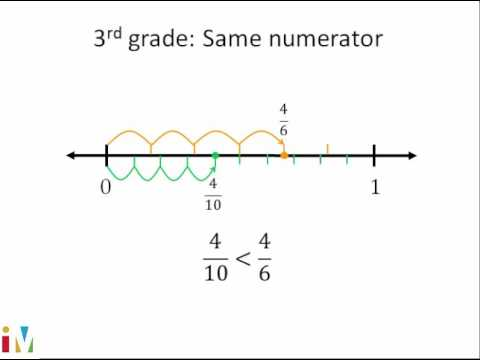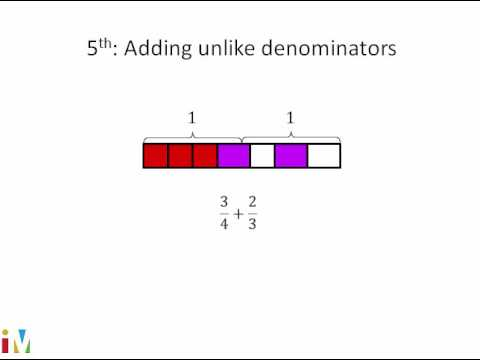# Creating equivalent fractions (Full video)

Description: Sal creates equivalent fractions by dividing a fraction model and number line into smaller parts. Created by Sal Khan. What I want you to do is pause this video and try to think of any other fractions that are the same that are equivalent to this fraction right over here that essentially represent the same number. Let me draw a whole, and I'm going to divide it into three equal sections. I'm drawing three equal sections.

### Other videos you might be interested in### Comparing Fractions

#### Illustrative Mathematics